# ShuffleNet-3D V1 Trained onVideo Datasets

Identify the main action in a video

Released in 2019, this family of nets consists of three-dimensional (3D) versions of the original ShuffleNet V1 architecture for video classification. The ShuffleNet V1 architecture utilizes pointwise group convolutions and channel shuffling, two new operations that greatly reduce computational cost while maintaining accuracy. With the availability of large-scale video datasets such as Jester and Kinetics-600, these models achieve much better accuracies compared to their two-dimensional counterparts for video classification task.

Number of models: 8

## Training Set Information

• Kinetics-600 dataset, containing six hundred human action classes with at least six hundred video clips for each action. For each class, there are also 50 and one hundred validation and test videos respectively. Jester dataset, containing 148,092 gesture videos under 27 classes.

## Performance

• The models achieve the following accuracies on the original ImageNet validation set.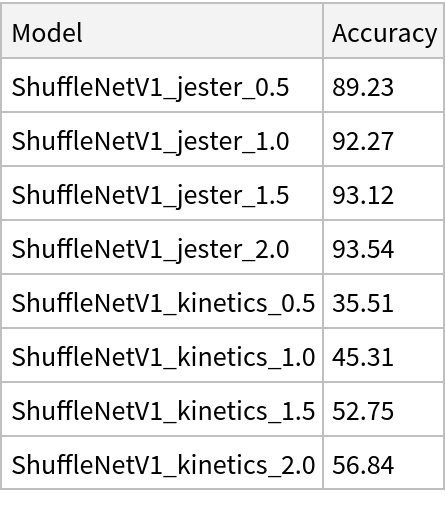## Examples

### Resource retrieval

Get the pre-trained net:

 In:=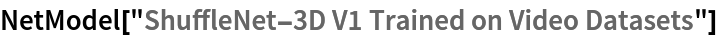Out=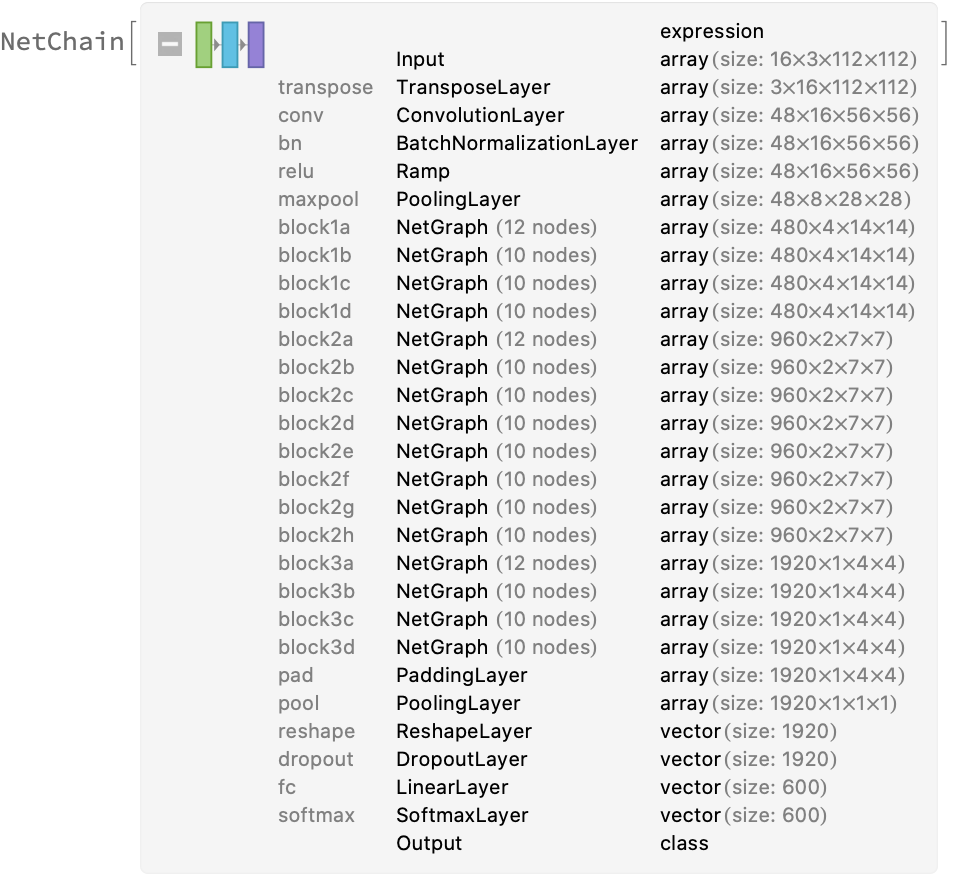### NetModel parameters

This model consists of a family of individual nets, each identified by a specific parameter combination. Inspect the available parameters:

 In:=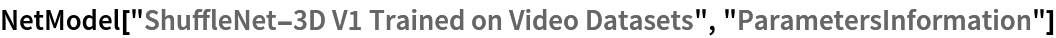Out=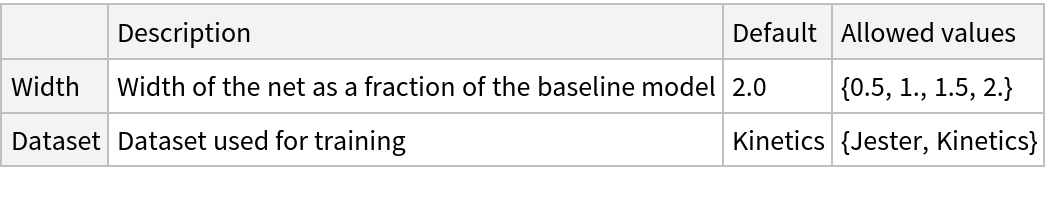Pick a non-default net by specifying the parameters:

 In:=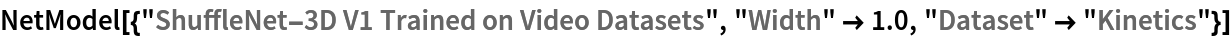Out=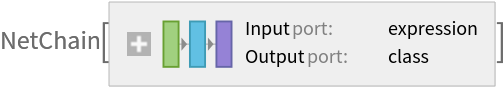Pick a non-default uninitialized net:

 In:=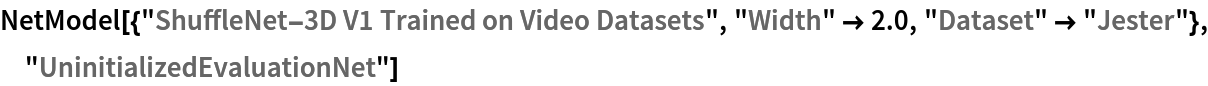Out=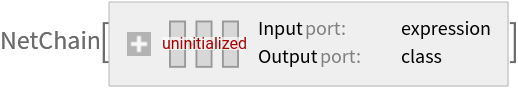### Basic usage

Identify the main action in a video:

 In:=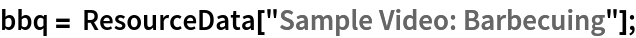In:=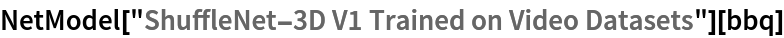Out=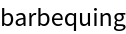Obtain the probabilities of the 10 most likely entities predicted by the net:

 In:=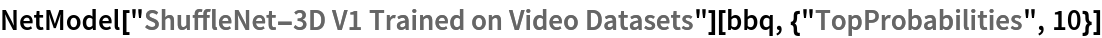Out=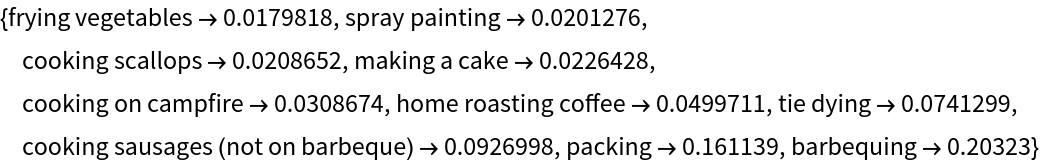Obtain the list of names of all available classes:

 In:=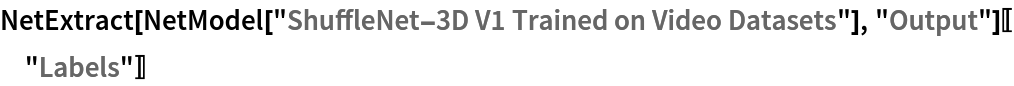Out=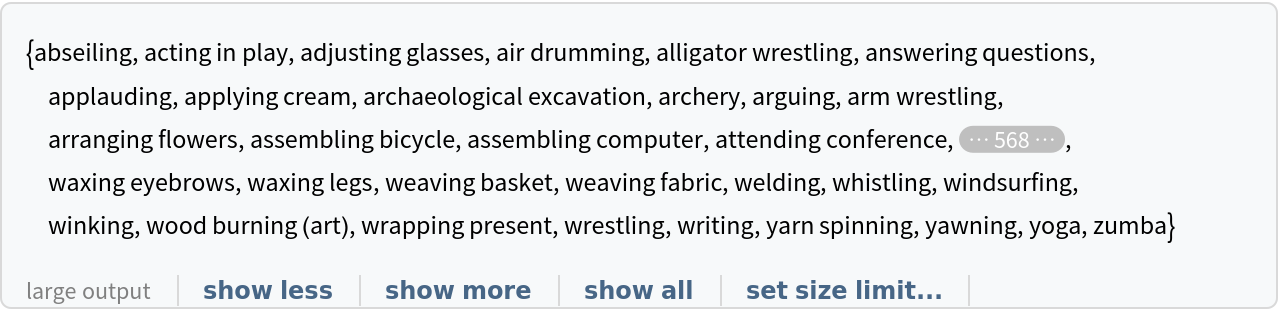### NetModel architecture

ShuffleNet-3D V1 features an efficient and elegant implementation of a channel shuffle operation: a feature map with gn channels is reshaped to expand the channel dimension to two dimensions of sizes (g, n), then transposed and further flattened back as the input of the next layer:

 In:=Out=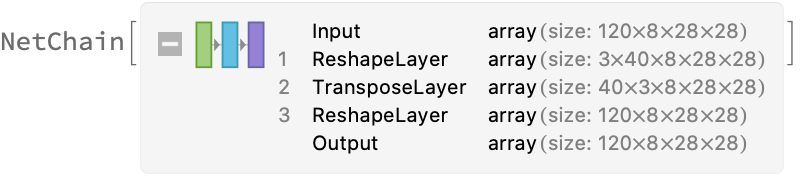In:=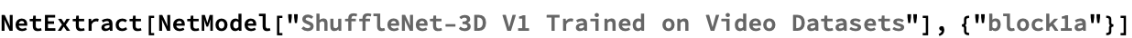Out=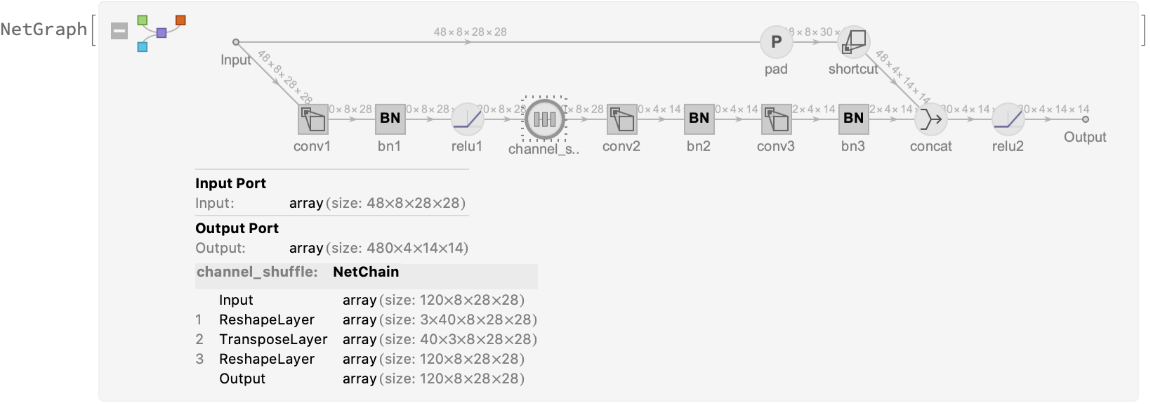### Feature extraction

Remove the last two layers of the trained net so that the net produces a vector representation of an image:

 In:=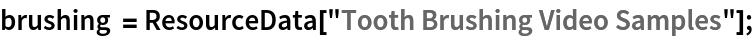In:=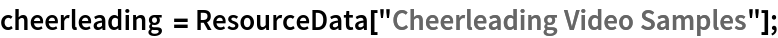In:=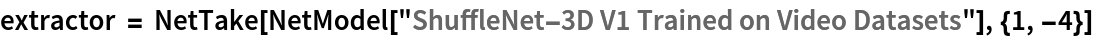Out=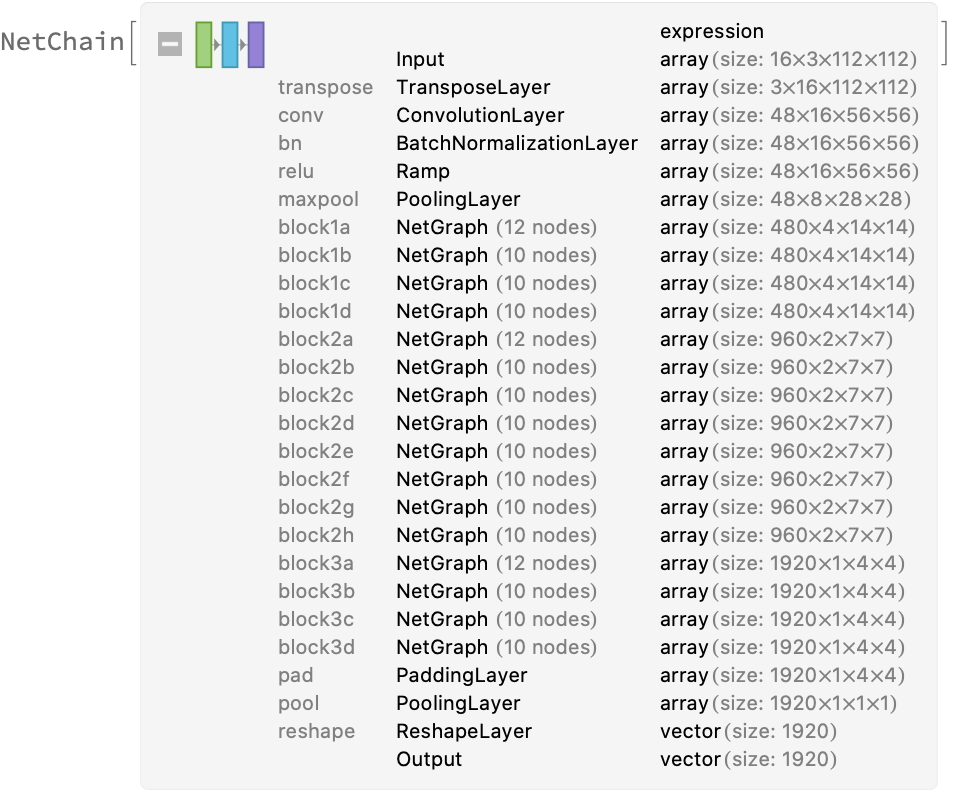Get a set of videos:

 In:=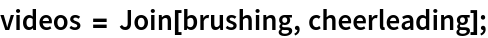Visualize the features of a set of videos:

 In:=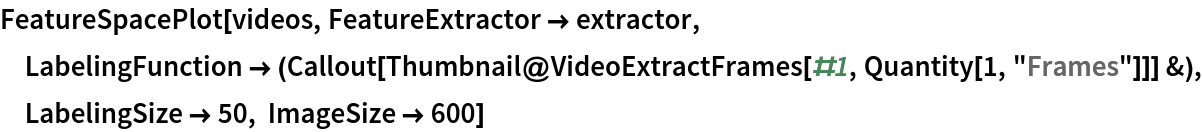Out=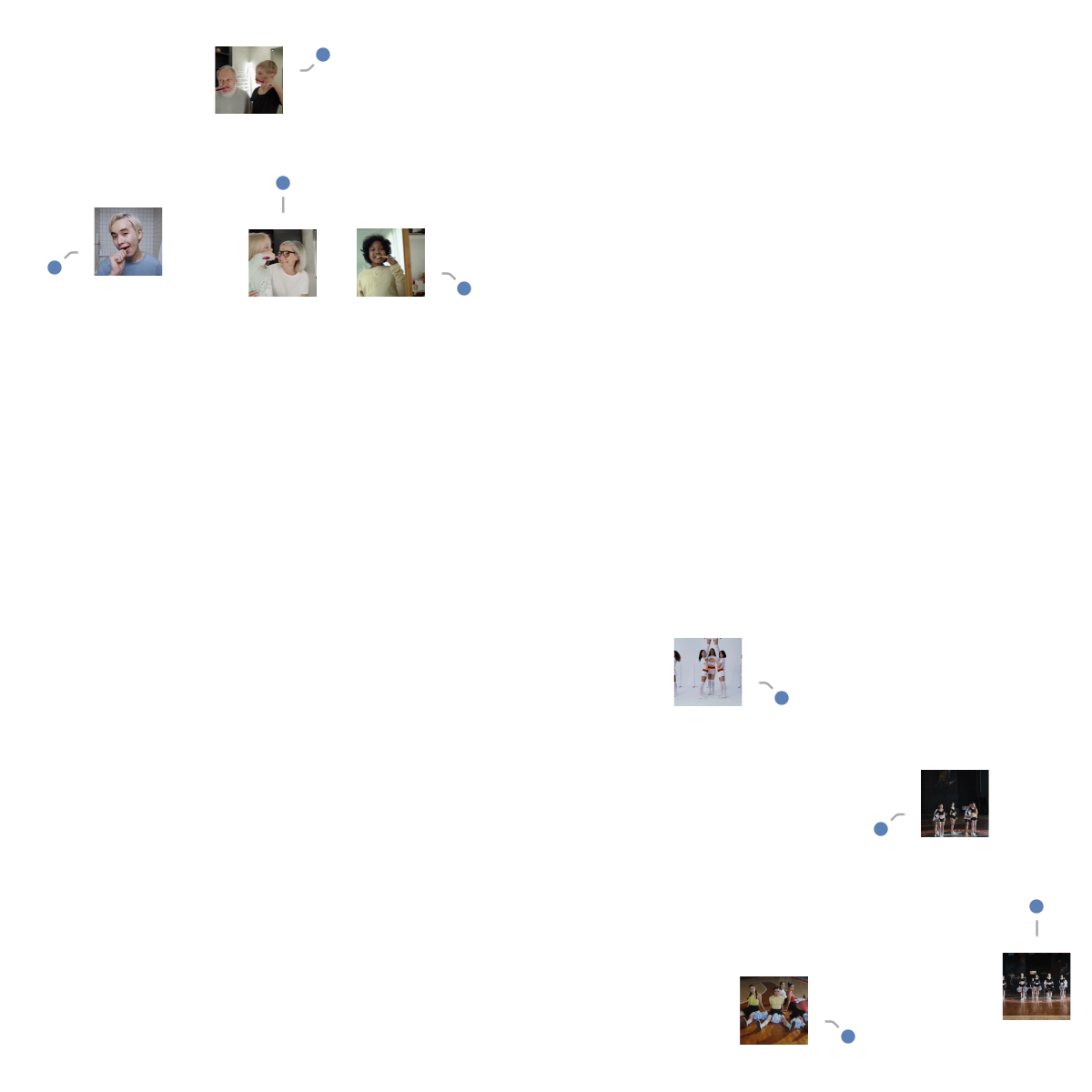### Transfer learning

Use the pre-trained model to build a classifier for telling apart images from two action classes not present in the dataset. Create a test set and a training set:

 In:=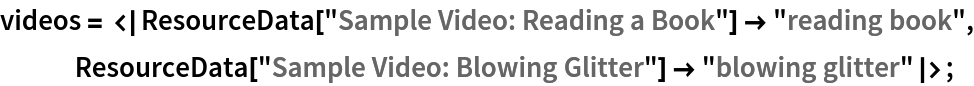In:=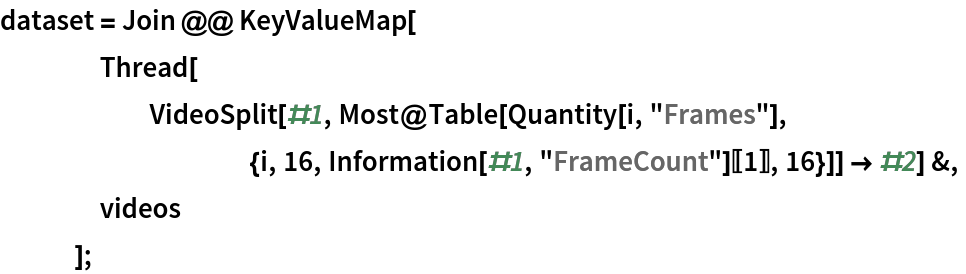In:=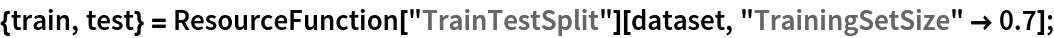Remove the linear layer from the pre-trained net:

 In:=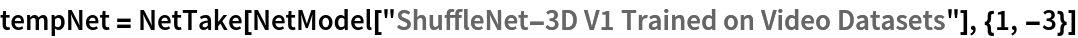Out=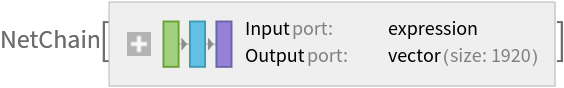Create a new net composed of the pre-trained net followed by a linear layer and a softmax layer:

 In:=Out=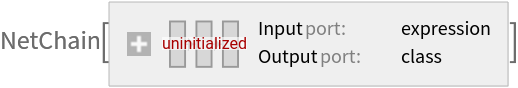Train on the dataset, freezing all the weights except for those in the "Linear" new layer (use TargetDevice -> "GPU" for training on a GPU):

 In:=Out=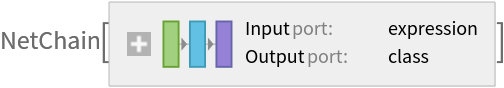Perfect accuracy is obtained on the test set:

 In:=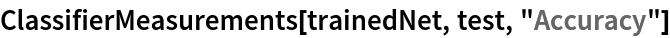Out=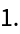### Net information

Inspect the number of parameters of all arrays in the net:

 In:=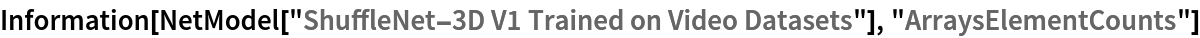Out=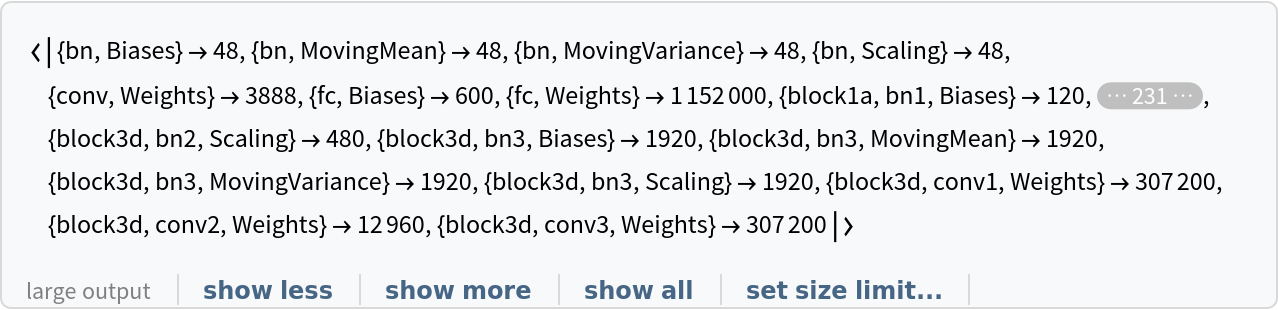Obtain the total number of parameters:

 In:=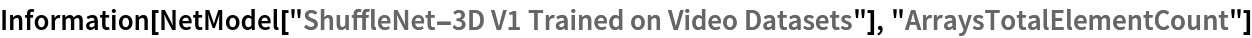Out=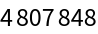Obtain the layer type counts:

 In:=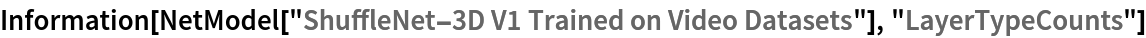Out=Display the summary graphic:

 In:=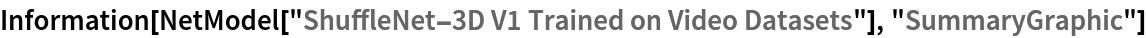Out=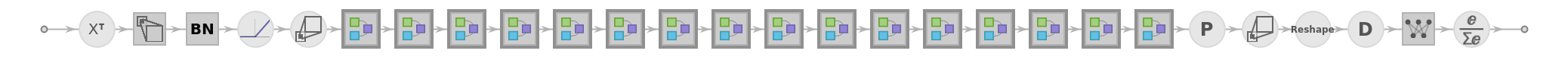### Export to ONNX

Export the net to the ONNX format:

 In:=Out=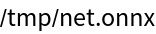Get the size of the ONNX file:

 In:=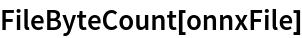Out=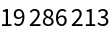Check some metadata of the ONNX model:

 In:=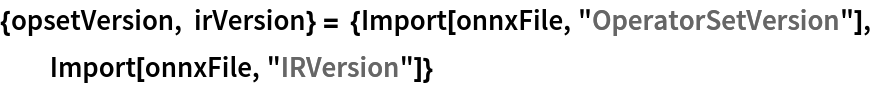Out=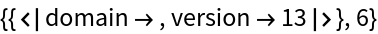Import the model back into the Wolfram Language. However, the NetEncoder and NetDecoder will be absent because they are not supported by ONNX:

 In:=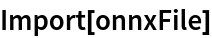Out=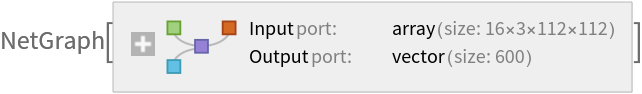## Reference

• O. Köpüklü, N. Kose, A. Gunduz, G. Rigoll, "Resource Efficient 3D Convolutional Neural Networks," arXiv:1904.02422 (2019)